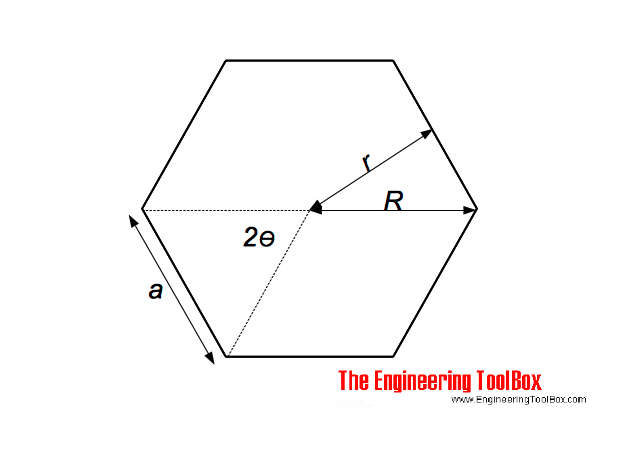Engineering ToolBox - Resources, Tools and Basic Information for Engineering and Design of Technical Applications!

# Regular Polygons

## Areas of regular polygons - polygons with 3 to 12 sides.### Perimeter of a Regular Polygon

P = n a                                 (1)

where

P = perimeter (m, ft ..)

n = number of sides

a = length of side (m, ft, ..)

### Area of a Regular Polygon

A = n a r / 2

= n r2 tan(Θ)

= n R2 sin(2 Θ) / 2                                (2)

Regular Polygons - Area vs. Number of Sides
PolygonNumber of Sides
- n -
Area
(m2, ft2 ..)
Triangle, equilateral 3 0.4330 a2
Square 4 1.0000 a2
Pentagon 5 1.7205 a2
Hexagon 6 2.5981 a2
Heptagon 7 3.6339 a2
Octagon 8 4.8284 a2
Nonagon 9 6.1818 a2
Decagon 10 7.6942 a2
Undecagon 11 9.3656 a2
Dodecagon 12 11.1961 a2

## Related Topics

• ### Mathematics

Mathematical rules and laws - numbers, areas, volumes, exponents, trigonometric functions and more.

## Related Documents

• ### Center of Gravity

A body and the center of gravity.
• ### Circle - the Chord Lengths when Divided in to Equal Segments

Calculate chord lengths when dividing the circumference of a circle into an equal number of segments.
• ### Hexagons and Squares - Diagonal Lengths

Distances between corners for hexagons and squares.
• ### Oblique Triangle

Calculate oblique triangles.
• ### Pythagorean Theorem

Verifying square corners.
• ### Right Angled Triangle

Right angled triangle equations.

Online vector calculator - add vectors with different magnitude and direction - like forces, velocities and more.

## Engineering ToolBox - SketchUp Extension - Online 3D modeling!

Add standard and customized parametric components - like flange beams, lumbers, piping, stairs and more - to your Sketchup model with the Engineering ToolBox - SketchUp Extension - enabled for use with older versions of the amazing SketchUp Make and the newer "up to date" SketchUp Pro . Add the Engineering ToolBox extension to your SketchUp Make/Pro from the Extension Warehouse !

We don't collect information from our users. More about

## Citation

• The Engineering ToolBox (2014). Regular Polygons. [online] Available at: https://www.engineeringtoolbox.com/regular-polygons-area-d_1872.html [Accessed Day Month Year].

Modify the access date according your visit.

9.19.12